Next: Derivation of van der Up: Quantum Statistics Previous: Quantum Statistics in Classical

# Quantum-Mechanical Treatment of Ideal Gas

Let us calculate the partition function of an ideal gas from quantum mechanics, making use of Maxwell-Boltzmann statistics. Obviously, such a partition function is only applicable when the gas is non-degenerate. According to Equations (8.67) and (8.69), we can write the partition function in the form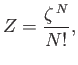(8.70)

where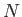is the number of constituent molecules,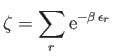(8.71)

is the partition function of an individual molecule, and the factor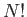is necessary to take into account the fact that the molecules are indistinguishable according to quantum theory.

Suppose that the gas is enclosed in a parallelepiped with sides of lengths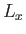,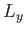, and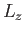. It follows that the de Broglie wavenumbers of the constituent molecules are quantized such that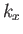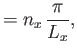(8.72)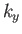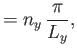(8.73)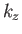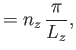(8.74)

where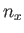,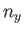, and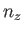are independent integers. (See Section C.10.) Thus, the allowed energy levels of a single molecule are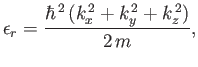(8.75)

where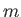is the molecular mass. Hence, we deduce that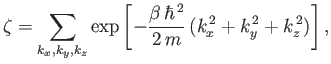(8.76)

where the sum is over all possible values of,,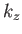. The previous expression can also be written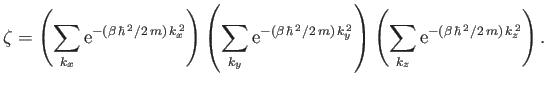(8.77)

Now, in the previous equation, the successive terms in the sum over(say) correspond to a very small increment,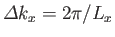, in(in fact, the increment can be made arbitrarily small by increasing), and, therefore, differ very little from each other. Hence, it is an excellent approximation to replace the sums in Equation (8.77) by integrals. Given that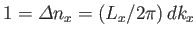, we can write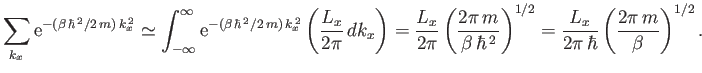(8.78)

(See Exercise 2.) Hence, Equation (8.77) becomes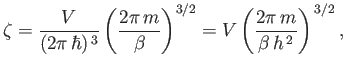(8.79)

where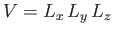is the volume of the gas.

It follows from Equation (8.70) that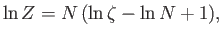(8.80)

where use has been made of Stirling's approximation. Thus, we obtain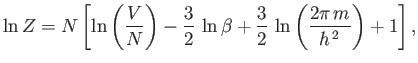(8.81)

and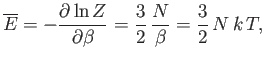(8.82)

and, finally,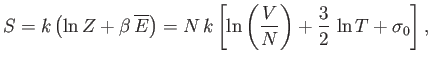(8.83)

where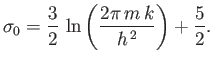(8.84)

These results are exactly the same as those obtained in Sections 7.7 and 7.8, using classical mechanics, except that the arbitrary parameter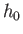is replaced by Planck's constant,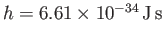.Next: Derivation of van der Up: Quantum Statistics Previous: Quantum Statistics in Classical
Richard Fitzpatrick 2016-01-25